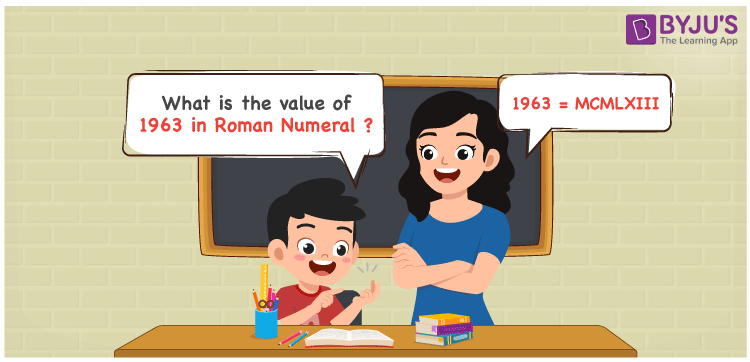# 1963 in Roman Numerals

1963 in Roman Numerals is MCMLXIII. Any Roman numeral uses only the 7 primary symbols which are I, V, X, L, C, D, and M, Where M represents 1000, C represents 100, L represents 50, X represents 10 and I represents 1. MCMLXIII represents the number 1963 which is obtained by the addition and subtraction rules of Roman Numerals. More on Rules to Write Roman Numerals can be read.

 Number Roman Numeral 1963 MCMLXIII

## How to Write 1963 in Roman Numerals?To convert 1963 in Roman Numerals, we need to represent 1963 as the sum of the fundamental symbols. 1963 can be broken down as

1. The number 1000,
2. Difference of 1000 and 100 to get 900
3. Sum of 50 and 10 to get 60
4. Sum of 1, 3 times
5. Add all the numbers from previous steps.

Let us understand it with Numerical representation.

1963 = 1000 + 900 + 60 + 1 + 1 + 1

1963 = 1000 + (1000 – 100) + (50 + 10) + 1 + 1 + 1

1963 = M + (M – C) + (L + X) + III

1963 = M + (CM) + LX + III

1963 = MCMLXIII.

## Video Lesson on Roman Numerals## Frequently Asked Questions on 1963 in Roman Numerals

Q1

### How to write the number 1963 in Roman Numerals?

1963 in Roman Numerals is written as MCMLXIII.
Q2

### How to read the Roman numerals MCMLXIII in English?

The Roman Numeral MCMLXIII represents the number 1963 and it is read as One Thousand Nine Hundred and Sixty Three.
Q3

### What is the year 1963 in Roman numerals?

The year 1963 in Roman Numerals is MCMLXIII.